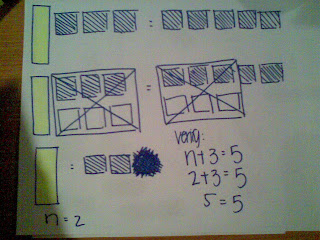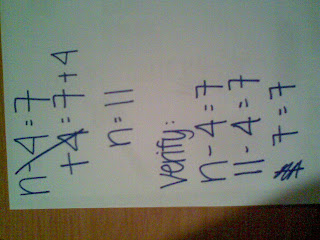### The Great Big Book Of Algebra

Thursday, December 4, 2008
Diamante - Adding Integers:

combining , increasing , adding
plus , add , more , gain
decreasing , reducing , diminishing
subtracted , reduced
Subtracting integers.

Haiku - Subtracting Integers:

Don't ever subtract
impossible to subtract

Free Verse - Ron's Rule:

Ron's rule works so good.
it helps you in tests just like it should.
you learn a lot just with this rule.
if you use it right you'll feel so cool.
It is a very smart rule to use.
once you use it you just cant refuse

Free Verse - Quotative:

This division is easy as pie,
All you have to do divide!
Divide by putting a number into a group
and see how much times it will fit!

Haiku - Partative:

Divide, share, give out
It's just like a deck of cards
Evenly sharing

Chapter 2: Combining Like Terms and Distributive Property

Leah: Hey Bella! Can you help me with my math homework?
Bella: Sure thing, which question do you need help on?
Leah: Number 5. The question was n+3-5n+12
Bella: Oh, that was easy!
Leah: No not really, I tried it but I'm not sure if I got it right or not
Leah: My answer turned out to be -6n+15
Bella: What?! My answer was -4n+15
Leah: Oh, I must have made a mistake. I will change it.
Bella: NO, you must know how to fix your mistakes! You see, you circle the two like terms which was n and -5n. After you must put it in order, -5n+n+3+12.
Leah: Yeah, I did put it in order ...
Bella: I know, but I'm not done yet. When you tried to solve -5n+n, you thought that it was -6n. You see, the term n by itself is one term. So, you must solve it like an integer question.
Leah: Ohh, I see now. So my mistake was that I added the n to-5 which made my answer -6n.
Bella: That's correct! You see, combining like terms!
Leah: Thanks Bella.
Bella: No problem! You see, if I never explained this to you then you would of not learn anything
Leah: Yes I know. Thanks again Bella, But I have another homework question I couldn't figure out.
Bella: No problem what question was it?
Leah: Number10. The question was 2 + 4(3n+8). And my answer was 12n + 10.
Bella: Oh! That question.
Leah: Yes that question. And this time, please explain to me what I did wrong.
Bella: Well the answer that I got was 12n+34. The thing that you did wrong was that you didnt multiply the 4 and 8 together. When ever theres brackets, you must multiply both numbers to the same number outside of the bracket.
Leah: Ohh, I get it now.
Bella: No problem! All you have to do is the distibutive property that we did in class the other day.
Leah: That was the thing that I was missing! Thanks alot Bella!
Bella: No problem! Just come to me next time if you have trouble on the other homework that we will have in future.
Leah: I will keep that in mind.

Here is my xtranormal movie.

Chapter 3: One Step Equation Solving
FIRST RULE before doing ANY algebraic equations. You MUST:
Isolate
Cancel Opposite
Balance
VerifySo this is an additive equation. To solve this equation you must Isolate the variable by adding the constants opposite. So in this algebraic equation, the constant is 3 so you have to add its opposite which is -3. What you do to the left side, you must do to the right side to balance it out. So its 5-3. Now on the left side, +3 and -3 make zero so you left with N the variable. Then you must solve the right side, 5-3 = 2. The answer would be N = 2. After you know what the variable equals, then you must verify which is just replacing the N with what it equals.Now using algebra tiles is pretty much the same thing but you must draw. The variable is the long yellow block ( sorry, I couldn't find any green or red markers/pencil crayons in my house) and the coloured square blocks are the constants. I started off with one yellow block = n, 3 blue squares = 3, then the answer which is 5 blue squares. Then you must Isolate the variable like always by adding the constants opposite. Then you Balance it out. So the answer is N=2. Then you Verify.

SUBTRACTIVE:When doing subtractive equations, you do the same steps when doing additive equations but just opposite. Also goes with the algebra tiles. So the equation is n-4=7. First you Isolate the variable so its n-4+4=7. Now Balance it out , 7+4. Now -4 and +4 equals zero and 7+4 = 11. So, n = 11. Now verify. First re-write the equation, n-4=7. Then what does n equal? 11. So 11-4=7. Then its 7=7. Whenever you verify, you must get the same answer as what the equation equals to.

MULTIPLICATIVE:The way I like to solve this multiplicative equations is using algebra tiles. First I drew 2 variables and 6 constants. Then you must divide it evenly like a deck of cards. After you divide it evenly like a deck of cards, then you must circle each group. So n = 3. Then you verify. 2n=6. 2(3)=6. 6=6.

DIVISIVE:I solved this equation by drawing the question. I drew one variable, and wrote down the fraction line and numbers. After I drew everything, I multiplied the answer which is 3 by 4. This is how you isolate when you are working on a divisive equation. So I multipied the 3 and 4 together and got 12. N = 12. Now you verify by re-writing the equation and replacing the n by what it equals to.

Chapter 4: Algetile Video
Here's mine and Sutchais Algetile Video. ENJOY! :)

1. alyannaL 8-17 said...

BALE ! 8-) Looks like I'm first. Anyways good job on your poems. You have some nice use of colors. My favorite poem you made is the Free Verse one about Ron's Rule. Well I dont you have any mistakes. Except I think you forgot to type "is" in "All you have to do divide!" In the middle of do and divide. Anyways good job ! :)

December 6, 2008 at 5:19 PM

2. peachy 8-41 said...

OHOH. NICE ADDING POEM! The one that switches! That ones pretty cool. I never did it, I thought it would be hard. :P So, I guess, way to be brave! :D AHHA. Nice Ron's Rule Poem! I liked how it rhymed. Good job!

December 7, 2008 at 8:42 PM

3. Leo-73 said...

Good job Michelle, I know where some of these poems came from. Anyway, good job, I liked Ron's Rule. It rhymed so much. Good job!

December 7, 2008 at 10:07 PM

4. sutchaisana 8-41 said...

GOOD JOB! YOU have nice rhyming skills. -__-"

December 9, 2008 at 12:00 AM

5. gelli 8-41 said...

GOOD JOB MICHELLE! (: You did an excellent job on showing how you solved each equation correctly. I love how you did your pictures! They were done so neatly! The algebra movie you did with Sutchai was done very well! You guys explained everything clearly that I could understand every step on how to solve it in algebra tiles. Keep up the good work and good job once again! (;

January 20, 2009 at 5:24 PM

6. ochoa 8-41 said...

great job baale! haa . nice colors you chose and great pictures . hahahaa, yea sutchais right! your so good at rhyming. the way you explained the pictures really helped!

February 11, 2009 at 8:23 PM